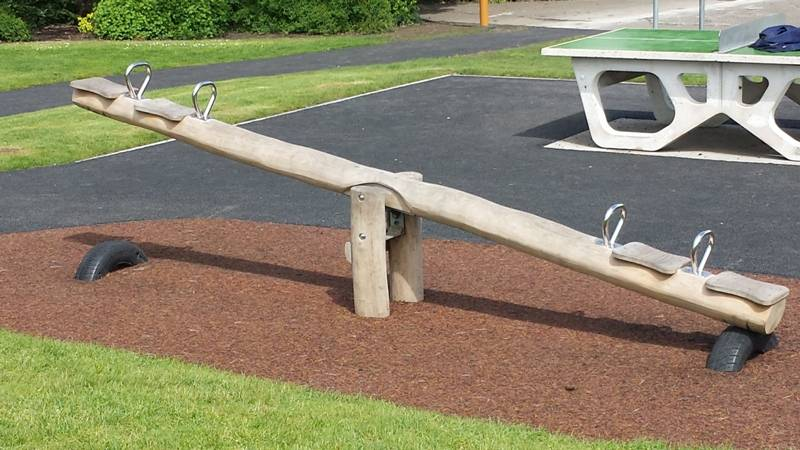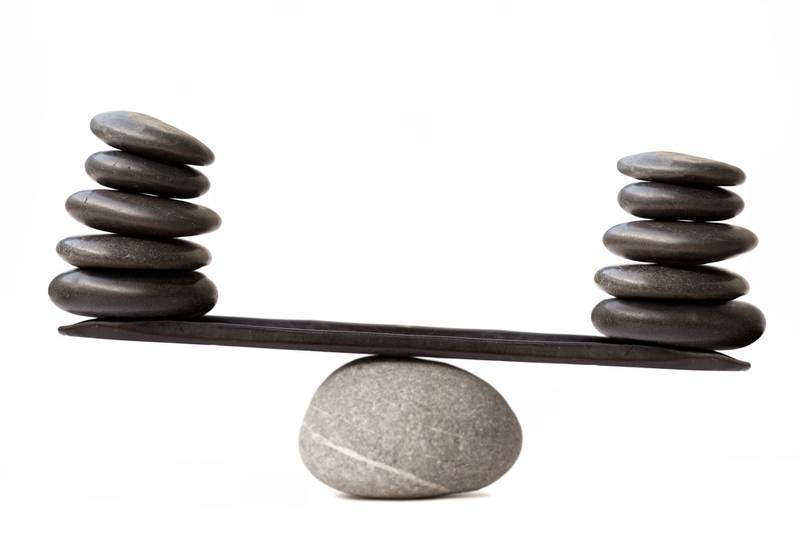# Perpetual motion machine - Intuitive meaning

CWatters
Homework Helper
Gold Member
Furthermore, this is an unstable equilibrium point, since if i put a feather on one end of the balanced lever, this end starts to go down until it's stopped by the ground. And this should happen in the lever system described by Mr. Feynman. Isn't it ?

Sure. It doesn't make a difference if you add weight to one side (you) or remove it from the other (Feyman).

•Aleoa
Sure. It doesn't make a difference if you add weight to one side (you) or remove it from the other (Feyman).

Thanks so much. So, in general, a balanced lever is parallel to the ground. Is it correct ?

berkeman
Mentor
Thanks so much. So, in general, a balanced lever is parallel to the ground. Is it correct ?
Why do you say that?#### Attachments

•jbriggs444
CWatters
Homework Helper
Gold Member
Thanks so much. So, in general, a balanced lever is parallel to the ground. Is it correct ?
No. I said it was balanced. So the net torque is zero. It could be at any angle.#### Attachments

•berkeman
CWatters
Homework Helper
Gold Member
Aleoa.. Can I check you understand that you understand something....

In the following I will assume there is no friction.

If the net torque on the balance is zero this means the balance is not accelerating. That does not mean the balance is stationary.

If the balance starts off stationary then it will remain stationary.

If the balance starts off rotating at a constant angular velocity it will continue rotating at a constant velocity. Even though it keeps rotating this does not mean it is a perpetual motion machine.

It's possible to make a machine that keeps rotating forever (eg two stars in orbit around each other) but this is not what scientists mean by a perpetual motion machine. To be a perpetual motion machine it must also be capable of doing some work even if that's small. Otherwise it is useless.

•Aleoa, berkeman and jbriggs444
Aleoa.. Can I check you understand that you understand something....

In the following I will assume there is no friction.

If the net torque on the balance is zero this means the balance is not accelerating. That does not mean the balance is stationary.

If the balance starts off stationary then it will remain stationary.

If the balance starts off rotating at a constant angular velocity it will continue rotating at a constant velocity. Even though it keeps rotating this does not mean it is a perpetual motion machine.

It's possible to make a machine that keeps rotating forever (eg two stars in orbit around each other) but this is not what scientists mean by a perpetual motion machine. To be a perpetual motion machine it must also be capable of doing some work even if that's small. Otherwise it is useless.

If i apply the formula of the moment , what i note is that a balanced lever is balanced in every possible angle of the lever with the ground. Is this true in the ideal case ?

CWatters
Homework Helper
Gold Member
If i apply the formula of the moment , what i note is that a balanced lever is balanced in every possible angle of the lever with the ground. Is this true in the ideal case ?
Yes.

•Aleoa
Yes.

And so, an ideal lever is reversible because, employing the same amount of energy used to change the state of the system i can go back to the initial state ?

CWatters
Homework Helper
Gold Member
No. If your system takes X units of energy to move in one direction and another X units to move it back then it will have consumed a total of 2X units of energy. So it's not reversible.

In a reversible system when it returns to the starting position/condition it will have consumed zero units of energy.

In the case for an ideal balance lever system it takes energy to get it moving (you give it KE) but you get that energy back when you stop it in the new position. So rotating it one way consumes zero energy and rotating it back again also consume zero energy. So it arrives back where it started having consumed zero energy. So an ideal balanced lever is a reversible system but not for the reason you gave.

Last edited:
...by its regenerative braking system.

It couldn't be in the same state anyway because of the brakes would generate heat because.

CWatters